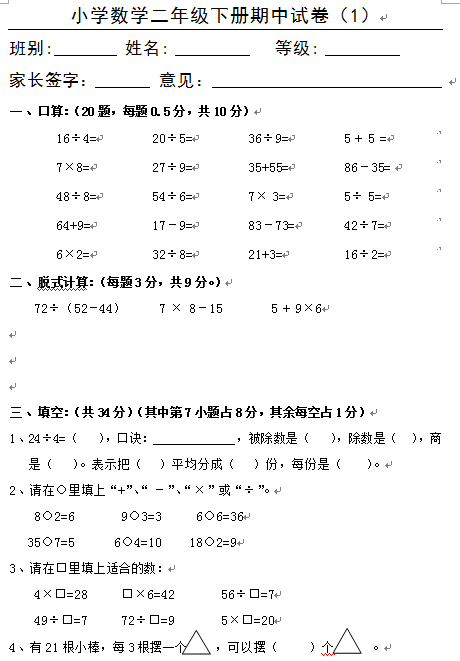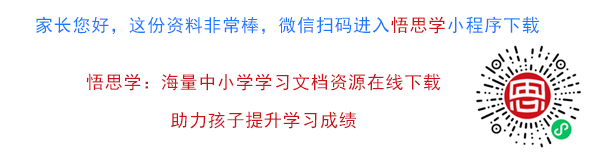16÷4= 20÷5= 36÷9= 5 + 5 = 7×8= 27÷9= 35+55= 86–35=

【DOC文档7页】数学小学二年级下册期中试卷A4电子版资料_可直接打印_会员免费下载##### 评论信息